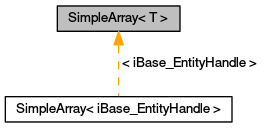MeshKit  1.0
SimpleArray< T > Class Template Reference

`#include <SimpleArray.hpp>`

Inheritance diagram for SimpleArray< T >:[legend]

List of all members.

## Public Types

typedef T * iterator
typedef const T * const_iterator

## Public Member Functions

SimpleArray ()
SimpleArray (unsigned s)
~SimpleArray ()
int size () const
int capacity () const
void resize (unsigned s)
void clear ()
void swap (SimpleArray &other)
iterator begin ()
const_iterator begin () const
iterator end ()
const_iterator end () const
T & operator[] (unsigned idx)
const T & operator[] (unsigned idx) const
T ** ptr_ ()
int * size_ ()
int * capacity_ ()

## Private Member Functions

SimpleArray (const SimpleArray &)
SimpleArrayoperator= (const SimpleArray &)

T * arr
int arrSize
int arrAllocated

## Detailed Description

### template<typename T> class SimpleArray< T >

Definition at line 8 of file SimpleArray.hpp.

## Member Typedef Documentation

 typedef const T* const_iterator

Definition at line 63 of file SimpleArray.hpp.

 typedef T* iterator

Definition at line 62 of file SimpleArray.hpp.

## Constructor & Destructor Documentation

 SimpleArray ( const SimpleArray< T > & ) ` [private]`
 SimpleArray ( ) ` [inline]`

Definition at line 19 of file SimpleArray.hpp.

 SimpleArray ( unsigned s ) ` [inline]`

Definition at line 21 of file SimpleArray.hpp.

 ~SimpleArray ( ) ` [inline]`

Definition at line 26 of file SimpleArray.hpp.

## Member Function Documentation

 iterator begin ( ) ` [inline]`

Definition at line 64 of file SimpleArray.hpp.

 const_iterator begin ( ) const` [inline]`

Definition at line 65 of file SimpleArray.hpp.

 int capacity ( ) const` [inline]`

Definition at line 32 of file SimpleArray.hpp.

 int* capacity_ ( ) ` [inline]`

Definition at line 75 of file SimpleArray.hpp.

 void clear ( ) ` [inline]`

Definition at line 44 of file SimpleArray.hpp.

 iterator end ( ) ` [inline]`

Definition at line 66 of file SimpleArray.hpp.

 const_iterator end ( ) const` [inline]`

Definition at line 67 of file SimpleArray.hpp.

 SimpleArray& operator= ( const SimpleArray< T > & ) ` [private]`
 T& operator[] ( unsigned idx ) ` [inline]`

Definition at line 69 of file SimpleArray.hpp.

 const T& operator[] ( unsigned idx ) const` [inline]`

Definition at line 70 of file SimpleArray.hpp.

 T** ptr_ ( ) ` [inline]`

Definition at line 73 of file SimpleArray.hpp.

 void resize ( unsigned s ) ` [inline]`

Definition at line 34 of file SimpleArray.hpp.

 int size ( ) const` [inline]`

Definition at line 31 of file SimpleArray.hpp.

 int* size_ ( ) ` [inline]`

Definition at line 74 of file SimpleArray.hpp.

 void swap ( SimpleArray< T > & other ) ` [inline]`

Definition at line 54 of file SimpleArray.hpp.

## Member Data Documentation

 T* arr` [private]`

Definition at line 15 of file SimpleArray.hpp.

 int arrAllocated` [private]`

Definition at line 17 of file SimpleArray.hpp.

 int arrSize` [private]`

Definition at line 16 of file SimpleArray.hpp.

The documentation for this class was generated from the following file: